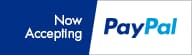Login Status Not logged in » Login# Resources

## Conversions

1 US gallon is equal to:
4 quarts128 ounces768 tsp
8 pints3.785 liters8.34 lbs
16 cups256 tbsp231 cu. in.
1 cup is equal to:
8 ounces48 tsp0.23 liter
1/2 pint236 ml14.4 cu.in.
16 tbsp0.52 lbs236 grams
1 tablespoon is equal to:
1/2 ounce3 tsp15 grams
15 ml180 dropso.663 cup
1 liter is equal to:
1000 ml4.328 cups202.88 tsp
2.164 pints67.63 tbsp0.264 gal
1000 grams2.22 lbs1.057 quarts
Measurement equivalents
3 tsp = 1 Tbsp1 ml = 1/5 tsp
2 Tbsp = 1 l uid oz5 ml = 1 tsp
16 Tbsp = 1 cup15 ml = 1 Tbsp
2 cups = 1 pint (16 oz)30 ml = 1 l uid oz
2 pints = 1 quart (32 oz)240 ml = 1 cup
4 quarts = 1 gal (128 oz)3840 ml = 1 gal
°F/°C TEMPERATURE CONVERSION
200°C = 392°F100°C = 212°F75°C = 167°F40°C = 104°F
150°C = 302°F95°C = 203°F70°C = 158°F30°C = 86°F
125°C = 257°F90°C = 194°F65°C = 149°F20°C = 68°F
110°C = 230°F85°C = 185°F60°C = 140°F10°C = 50°F
105°C = 221°F80°C = 176°F50°C = 122°F0°C = 32°F
°F/°C TEMPERATURE FORMULA
°C = (°F - 32) x 5/9°F = (°C x 9/5) + 32
Lighting Conversion
1 footcandle = lumen/ft2
Definitions & Abbreviations
ppm = parts per million
mho = conductivity
mS = millisiemen
uS = microsiemen
tds = total dissolved solids
ec = electrical conductivity
pH = potential hydrogen
Definitions & Abbreviations
C CarbonMn Mangenese
Ca CalciumMo Molybdenum
Cl ChlorineN Nitrogen
Cu CopperNa Sodium
Fe IronO Oxygen
F FluorineP Phosphorus
H HydrogenS Sulfur
K PotassiumZn Zinc
 1 Siemen = 1000 mS 1 mS = 1000 uS 1 mS = 500 ppm (standard) 1 mS = 650 ppm (horticultural) 1 mg/liter = 1 ppm